# chapter_14_chemical equilibrium.ppt

22 de Sep de 2022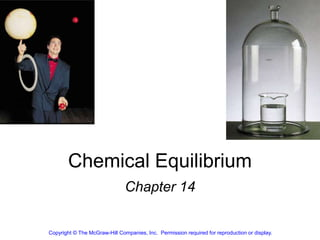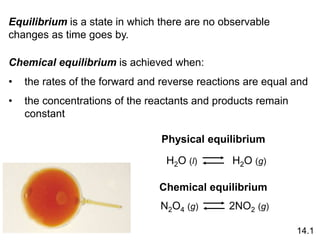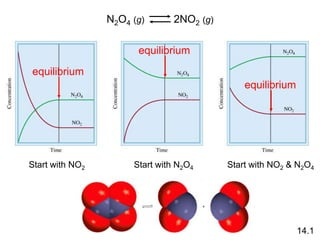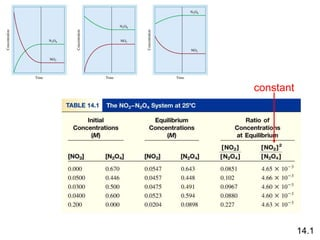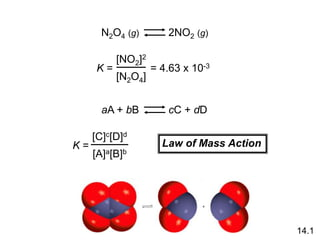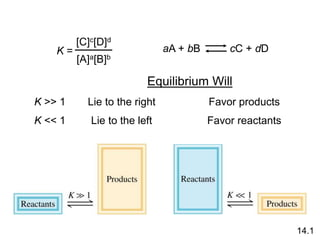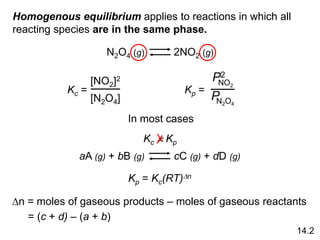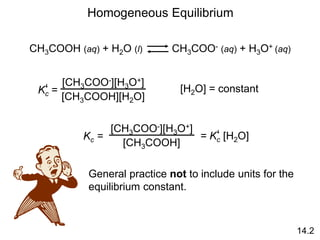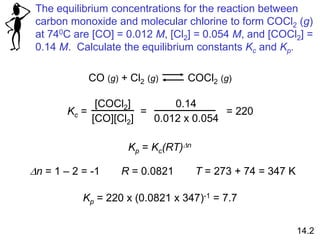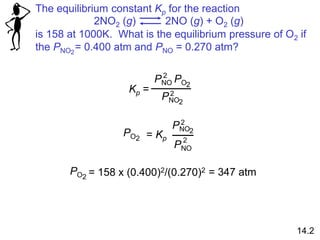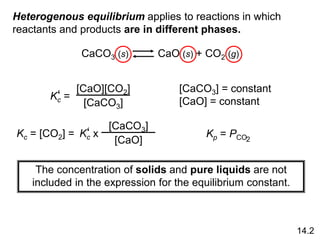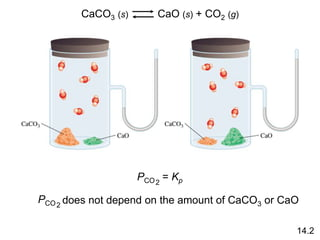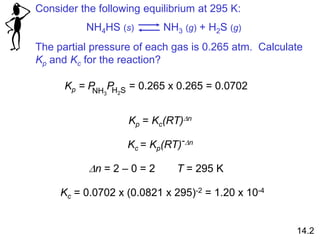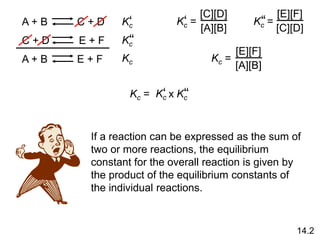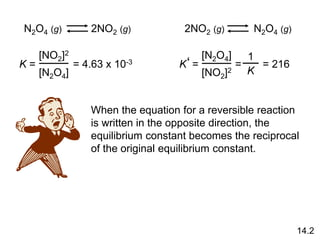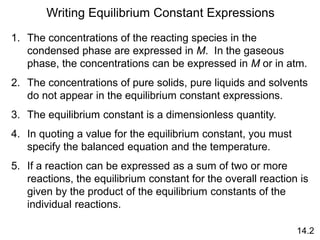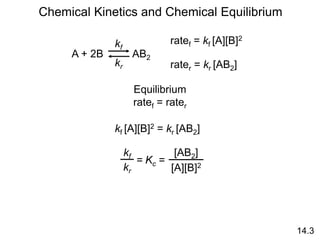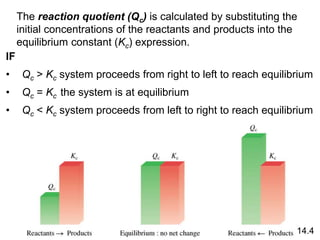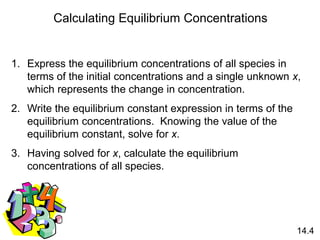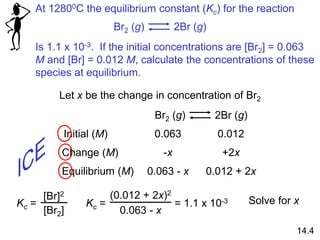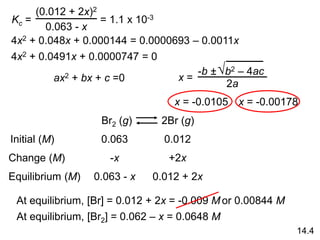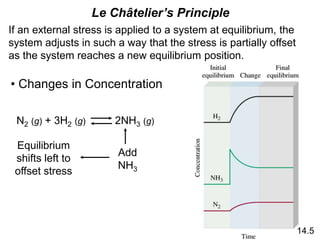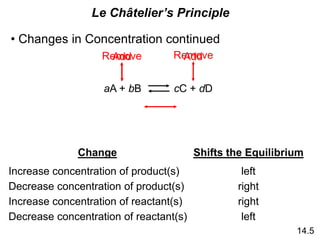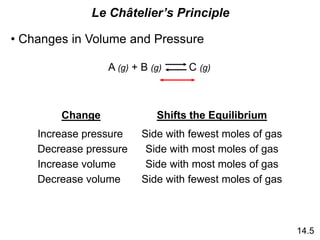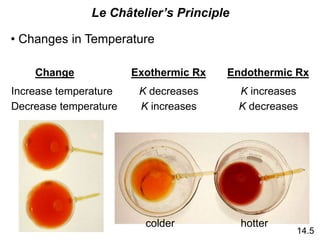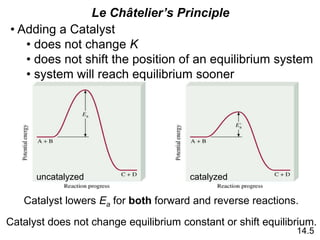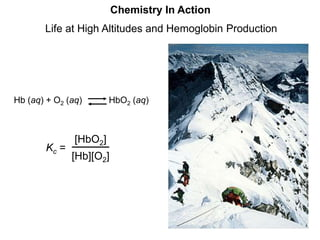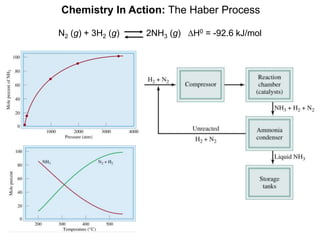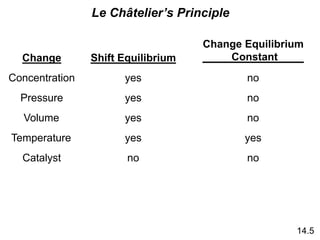1 de 29

### chapter_14_chemical equilibrium.ppt

• 1. Chemical Equilibrium Chapter 14 Copyright © The McGraw-Hill Companies, Inc. Permission required for reproduction or display.
• 2. Equilibrium is a state in which there are no observable changes as time goes by. Chemical equilibrium is achieved when: • the rates of the forward and reverse reactions are equal and • the concentrations of the reactants and products remain constant Physical equilibrium H2O (l) Chemical equilibrium N2O4 (g) 14.1 H2O (g) 2NO2 (g)
• 5. N2O4 (g) 2NO2 (g) = 4.63 x 10-3 K = [NO2]2 [N2O4] aA + bB cC + dD K = [C]c[D]d [A]a[B]b Law of Mass Action 14.1
• 6. K >> 1 K << 1 Lie to the right Favor products Lie to the left Favor reactants Equilibrium Will K = [C]c[D]d [A]a[B]b aA + bB cC + dD 14.1
• 7. Homogenous equilibrium applies to reactions in which all reacting species are in the same phase. N2O4 (g) 2NO2 (g) Kc = [NO2]2 [N2O4] Kp = NO2 P2 N2O4 P aA (g) + bB (g) cC (g) + dD (g) 14.2 Kp = Kc(RT)Dn Dn = moles of gaseous products – moles of gaseous reactants = (c + d) – (a + b) In most cases Kc  Kp
• 8. Homogeneous Equilibrium CH3COOH (aq) + H2O (l) CH3COO- (aq) + H3O+ (aq) Kc = ‘ [CH3COO-][H3O+] [CH3COOH][H2O] [H2O] = constant Kc = [CH3COO-][H3O+] [CH3COOH] = Kc [H2O] ‘ General practice not to include units for the equilibrium constant. 14.2
• 9. The equilibrium concentrations for the reaction between carbon monoxide and molecular chlorine to form COCl2 (g) at 740C are [CO] = 0.012 M, [Cl2] = 0.054 M, and [COCl2] = 0.14 M. Calculate the equilibrium constants Kc and Kp. CO (g) + Cl2 (g) COCl2 (g) Kc = [COCl2] [CO][Cl2] = 0.14 0.012 x 0.054 = 220 Kp = Kc(RT)Dn Dn = 1 – 2 = -1 R = 0.0821 T = 273 + 74 = 347 K Kp = 220 x (0.0821 x 347)-1 = 7.7 14.2
• 10. The equilibrium constant Kp for the reaction is 158 at 1000K. What is the equilibrium pressure of O2 if the PNO = 0.400 atm and PNO = 0.270 atm? 2 2NO2 (g) 2NO (g) + O2 (g) 14.2 Kp = 2 PNO PO 2 PNO 2 2 PO2 = Kp PNO 2 2 PNO 2 PO2 = 158 x (0.400)2/(0.270)2 = 347 atm
• 11. Heterogenous equilibrium applies to reactions in which reactants and products are in different phases. CaCO3 (s) CaO (s) + CO2 (g) Kc = ‘ [CaO][CO2] [CaCO3] [CaCO3] = constant [CaO] = constant Kc = [CO2] = Kc x ‘ [CaCO3] [CaO] Kp = PCO2 The concentration of solids and pure liquids are not included in the expression for the equilibrium constant. 14.2
• 12. PCO2 = Kp CaCO3 (s) CaO (s) + CO2 (g) PCO2 does not depend on the amount of CaCO3 or CaO 14.2
• 13. Consider the following equilibrium at 295 K: The partial pressure of each gas is 0.265 atm. Calculate Kp and Kc for the reaction? NH4HS (s) NH3 (g) + H2S (g) Kp = P NH3 H2S P = 0.265 x 0.265 = 0.0702 Kp = Kc(RT)Dn Kc = Kp(RT)-Dn Dn = 2 – 0 = 2 T = 295 K Kc = 0.0702 x (0.0821 x 295)-2 = 1.20 x 10-4 14.2
• 14. A + B C + D C + D E + F A + B E + F Kc = ‘ [C][D] [A][B] Kc = ‘‘ [E][F] [C][D] [E][F] [A][B] Kc = Kc ‘ Kc‘ ‘ Kc Kc = Kc‘ ‘ Kc ‘ x If a reaction can be expressed as the sum of two or more reactions, the equilibrium constant for the overall reaction is given by the product of the equilibrium constants of the individual reactions. 14.2
• 15. N2O4 (g) 2NO2 (g) = 4.63 x 10-3 K = [NO2]2 [N2O4] 2NO2 (g) N2O4 (g) K = [N2O4] [NO2]2 ‘ = 1 K = 216 When the equation for a reversible reaction is written in the opposite direction, the equilibrium constant becomes the reciprocal of the original equilibrium constant. 14.2
• 16. Writing Equilibrium Constant Expressions 1. The concentrations of the reacting species in the condensed phase are expressed in M. In the gaseous phase, the concentrations can be expressed in M or in atm. 2. The concentrations of pure solids, pure liquids and solvents do not appear in the equilibrium constant expressions. 3. The equilibrium constant is a dimensionless quantity. 4. In quoting a value for the equilibrium constant, you must specify the balanced equation and the temperature. 5. If a reaction can be expressed as a sum of two or more reactions, the equilibrium constant for the overall reaction is given by the product of the equilibrium constants of the individual reactions. 14.2
• 17. 14.3 Chemical Kinetics and Chemical Equilibrium A + 2B AB2 kf kr ratef = kf [A][B]2 rater = kr [AB2] Equilibrium ratef = rater kf [A][B]2 = kr [AB2] kf kr [AB2] [A][B]2 = Kc =
• 18. The reaction quotient (Qc) is calculated by substituting the initial concentrations of the reactants and products into the equilibrium constant (Kc) expression. IF • Qc > Kc system proceeds from right to left to reach equilibrium • Qc = Kc the system is at equilibrium • Qc < Kc system proceeds from left to right to reach equilibrium 14.4
• 19. Calculating Equilibrium Concentrations 1. Express the equilibrium concentrations of all species in terms of the initial concentrations and a single unknown x, which represents the change in concentration. 2. Write the equilibrium constant expression in terms of the equilibrium concentrations. Knowing the value of the equilibrium constant, solve for x. 3. Having solved for x, calculate the equilibrium concentrations of all species. 14.4
• 20. At 12800C the equilibrium constant (Kc) for the reaction Is 1.1 x 10-3. If the initial concentrations are [Br2] = 0.063 M and [Br] = 0.012 M, calculate the concentrations of these species at equilibrium. Br2 (g) 2Br (g) Br2 (g) 2Br (g) Let x be the change in concentration of Br2 Initial (M) Change (M) Equilibrium (M) 0.063 0.012 -x +2x 0.063 - x 0.012 + 2x [Br]2 [Br2] Kc = Kc = (0.012 + 2x)2 0.063 - x = 1.1 x 10-3 Solve for x 14.4
• 21. Kc = (0.012 + 2x)2 0.063 - x = 1.1 x 10-3 4x2 + 0.048x + 0.000144 = 0.0000693 – 0.0011x 4x2 + 0.0491x + 0.0000747 = 0 ax2 + bx + c =0 -b ± b2 – 4ac  2a x = Br2 (g) 2Br (g) Initial (M) Change (M) Equilibrium (M) 0.063 0.012 -x +2x 0.063 - x 0.012 + 2x x = -0.00178 x = -0.0105 At equilibrium, [Br] = 0.012 + 2x = -0.009 M or 0.00844 M At equilibrium, [Br2] = 0.062 – x = 0.0648 M 14.4
• 22. If an external stress is applied to a system at equilibrium, the system adjusts in such a way that the stress is partially offset as the system reaches a new equilibrium position. Le Châtelier’s Principle • Changes in Concentration N2 (g) + 3H2 (g) 2NH3 (g) Add NH3 Equilibrium shifts left to offset stress 14.5
• 23. Le Châtelier’s Principle • Changes in Concentration continued Change Shifts the Equilibrium Increase concentration of product(s) left Decrease concentration of product(s) right Decrease concentration of reactant(s) Increase concentration of reactant(s) right left 14.5 aA + bB cC + dD Add Add Remove Remove
• 24. Le Châtelier’s Principle • Changes in Volume and Pressure A (g) + B (g) C (g) Change Shifts the Equilibrium Increase pressure Side with fewest moles of gas Decrease pressure Side with most moles of gas Decrease volume Increase volume Side with most moles of gas Side with fewest moles of gas 14.5
• 25. Le Châtelier’s Principle • Changes in Temperature Change Exothermic Rx Increase temperature K decreases Decrease temperature K increases Endothermic Rx K increases K decreases 14.5 colder hotter
• 26. uncatalyzed catalyzed 14.5 Catalyst lowers Ea for both forward and reverse reactions. Catalyst does not change equilibrium constant or shift equilibrium. • Adding a Catalyst • does not change K • does not shift the position of an equilibrium system • system will reach equilibrium sooner Le Châtelier’s Principle
• 27. Chemistry In Action Life at High Altitudes and Hemoglobin Production Kc = [HbO2] [Hb][O2] Hb (aq) + O2 (aq) HbO2 (aq)
• 28. Chemistry In Action: The Haber Process N2 (g) + 3H2 (g) 2NH3 (g) DH0 = -92.6 kJ/mol
• 29. Le Châtelier’s Principle Change Shift Equilibrium Change Equilibrium Constant Concentration yes no Pressure yes no Volume yes no Temperature yes yes Catalyst no no 14.5